This defines a function pinks that takes any argument:
 In:=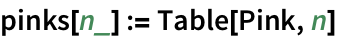This uses the function definition:
 In:=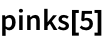Out=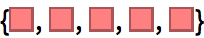In:=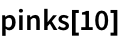Out=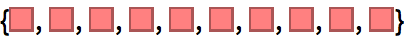How does this function definition work? The idea is that the := defines a value for the pattern pinks[n_]. When you ask for pinks, this matches the pinks[n_] pattern, and the value youve defined for this is used.
But this is just the beginning of the story of function definition in the Wolfram Language. Because in the Wolfram Language, you can give a definition for anything.
Heres a list of expressions:
 In:=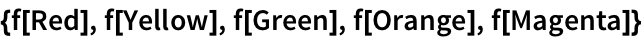Out=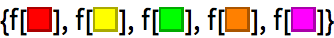Define values for f[Red] and f[Green]:
 In:=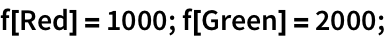Now f[Red] and f[Green] are replaced by the values defined; the other cases are left unchanged:
 In:=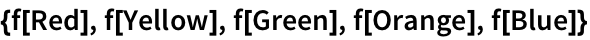Out=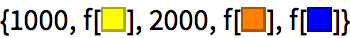Now lets add a definition for the pattern f[x_]. The Wolfram Language will use this whenever the special definitions for f[Red] and f[Green] dont apply.
Define a value for f with any argument:
 In:=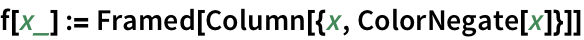In:=Out=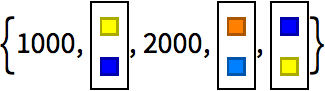In:=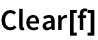Give a recursive definition of factorial:
 In:=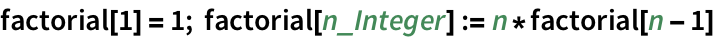In:=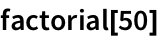Out=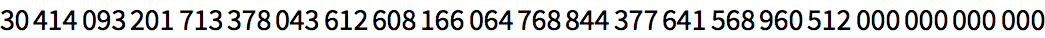In:=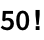Out=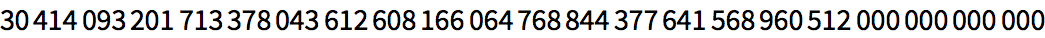Instead of having definitions for factorial and factorial[n_] we could have had a single definition and used If. But having separate definitions for each case tends to make things much easier to read and understand.
An alternative definition using If:
 In:=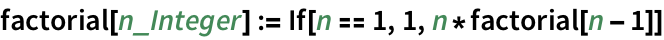Its nice to be able to break out special cases, but the real power of being able to make definitions for anything comes when one goes beyond simple function[arguments] cases.
As a simple example, consider making a definition for plusminus[{x_,y_}].
Define a value for a pattern:
 In:=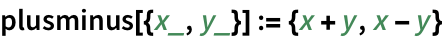Use the definition:
 In:=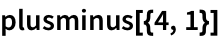Out=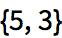In:=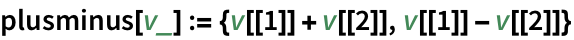Its very common to want to define a function that applies only to objects with a certain structure. This is easy to do with patterns. Heres an example.
 In:=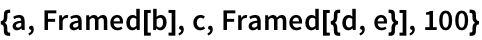Out=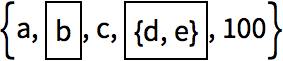Define a function that applies only to framed objects:
 In:=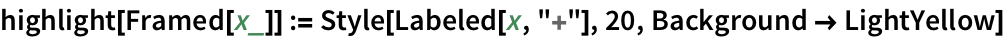Apply highlight to each element of a list; it knows what to do when its given something framed:
 In:=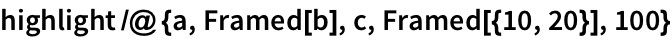Out=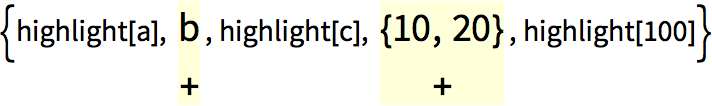This definition applies to anything with head List:
 In:=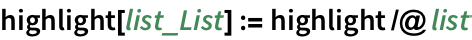Now you no longer have to use /@:
 In:=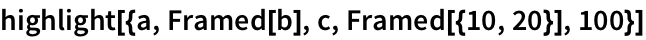Out=Give a general case, to use if none of the special cases apply:
 In:=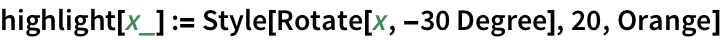This uses the special cases when it can, then the general case when nothing else applies:
 In:=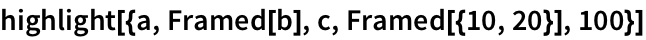Out=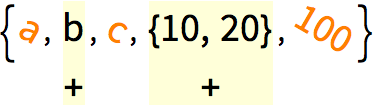Note: These exercises involve defining functions. Remember to use Clear to get rid of definitions once youre finished with each exercise.
40.1Define a function f that computes the square of its argument. »
No expected output
Many possible solutions of the form _:=_ or _=_Answer & check your solution
40.2Define a function poly that takes an integer, and makes a picture of an orange regular polygon with that number of sides. »
No expected output
Many possible solutions of the form _:=_ or _=_Answer & check your solution
40.3Define a function f that takes a list of two elements and puts them in reverse order. »
No expected output
Many possible solutions of the form _:=_ or _=_Answer & check your solution
40.4Create a function f that takes two arguments and gives the result of multiplying them and dividing by their sum. »
No expected output
Many possible solutions of the form _:=_ or _=_Answer & check your solution
40.5Define a function f that takes a list of two elements and returns a list of their sum, difference and ratio. »
No expected output
Many possible solutions of the form _:=_ or _=_Answer & check your solution
40.6Define a function evenodd that gives Black if its argument is even and White otherwise, but gives Red if its argument is 0. »
No expected output
Many possible solutions of the form _:=_ or _=_Answer & check your solution
40.7Define a function f of three arguments where the second two arguments are added if the first argument is 1, multiplied if its 2 and raised to a power if its 3. »
No expected output
Many possible solutions of the form _:=_ or _=_Answer & check your solution
40.8Define a Fibonacci function f with f and f both being 1, and f[n] for integer n being the sum of f[n-1] and f[n-2]»
No expected output
Many possible solutions of the form _:=_ or _=_Answer & check your solution
40.9Create a function animal that takes a string, and gives a picture of an animal with that name. »
No expected output
Many possible solutions of the form _:=_ or _=_Answer & check your solution
40.10Define a function nearwords that takes a string and an integer n, and gives the n words in WordList[ ] that are nearest to a given string. »
No expected output
Many possible solutions of the form _:=_ or _=_Answer & check your solution
What kind of a pattern can be used in a function definition?
Absolutely any pattern you want. Even one where the head is itself a pattern.
Use ?f to see the definitions for f.
How do I overwrite an existing function definition?
How are different definitions for a particular function sorted?
Typically from most specific to least specific. If there are definitions that cant be ordered by specificity, definitions made later are put later. When definitions are used, the earlier ones are tried first. ?f shows the ordering of definitions for f.
Can I redefine built-in functions like Max or Plus?
Usually yes. First, though, you often have to say e.g. Unprotect[Max]. Then definitions you add will be used in preference to built-in ones. Some functions, like Plus, are so fundamental that the system locks them in a protected state. Even in this case, though, you can make upvalue definitions that are associated with particular structures of arguments.
Can I do object-oriented programming in the Wolfram Language?
Can I use = instead of := for function definitions?
Sometimes. f[n_]=n^2 will work fine, because the right-hand side doesnt evaluate when you make the assignment. f[n_]=Now and f[n_]:=Now will give different results. And in many cases the right-hand side cant be meaningfully evaluated until specific arguments are given.
How can I share function definitions with other people?
Just send the code! A convenient way to do this through the cloud is to use CloudSave and CloudGet, as discussed in Section 43.